# Probability

## Combinatorics

### Example 15

Ex:            Calculate the odds (or probabilities) of the following 5-card poker hands:

Also, verify that the probabilities sum to unity. Assume a 52-card deck. (The number of possible 5-card hands is 52C5 = 2,598,960.)

Sol'n:  a)  A royal flush is ace, king, queen, jack, and ten of the same suit.

If we order the 5-card hand from highest card to lowest, the first card will be an ace. There are four possible suits for the ace. After that, the other four cards are completely determined. Thus, there are 4 possible royal flushes:

# royal flushes = 4C1 = 4

Dividing by the number of possible hands gives the probability: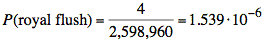or 1 in 649,740

b) A straight-flush (excluding royal flush) is all cards the same suit and showing consecutive numbers (but not the highest 5 consecutive numbers).

If we order the 5-card hand from highest number to lowest, the first card may be one of the following: king, queen, jack, 10, 9, 8, 7, 6, or 5. (Note: the ace may be the card above a king or below a 2, but we would have a royal flush if it were the card above the king.) There are 9 possibilities. After the first card, whose suit we may choose in 4 ways, the remaining cards are completely determined.

# straight flushes = 9 4C1 = 9⋅4 = 36

Subtracting the number of royal flushes and dividing by the number of possible hands gives the probability: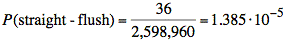or 1 in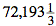.

c)  A four-of-a-kind is four cards showing the same number plus any other card.

If we order the 5-card hand with the four-of-a-kind first, we have 13C1 choices for the number showing on the first four cards. Since we will have all four suits, we have only 4C4 = 1 way to choose the suits. The remaining card will be any of the 48 remaining cards:

# 4-of-a-kinds = 13C14C448C1 = 13⋅1⋅48 = 624

Dividing by the number of possible hands gives the probability: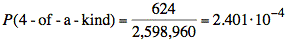or 1 in 4165

d) A full house is three cards showing the same number plus a pair.

If we order the 5-card hand with the three-of-a-kind first, we have 13C1 choices for the number showing on the first three cards. For the choice of suits, we have three out of four possible suits or 4C3 = 4 possibilities. For the remaining pair, we have 12C1 choices for the number showing on the two cards. For the choice of suits, we have two out of four possible suits or 4C2 = 6 possibilities.

# full houses = 13C14C312C14C2 = 13⋅4⋅12⋅6 = 3744

Dividing by the number of possible hands gives the probability: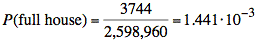or 1 in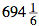e)  A flush (excluding straight-flush) is all cards the same suit (but not a straight).

If we pick the suit first, we have 4C1 = 4 choices. For that suit, there are 13 cards from which we choose 5. Thus, we have 13C5 = 1287 choices. Subtracting the straights, (which may start with ace, king, queen, ... 5), reduces this number by 10.

# flushes (not straight) = 4C1⋅(13C5 − 10) = 4⋅1277 = 5108

Dividing by the number of possible hands gives the probability: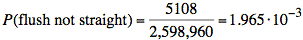or 1 in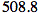f)  A straight (excluding straight-flush) is five cards showing consecutive numbers (but not all of the same suit).

If we order the 5-card hand from highest number to lowest, the first card may be one of the following: ace, king, queen, jack, 10, 9, 8, 7, 6, or 5. (Note: the ace may be the card above a king or below a 2.) There are 10 possibilities. After the first card, the numbers showing on the remaining four cards are completely determine. If we allow flushes, including royal flushes, there are four possible suits for each of the cards. (Note that this holds because the cards all show different numbers, and there are four suits for each number.)

# straights = 10⋅4C14C14C14C14C1 = 10⋅45 = 10,240

Subtracting the number of straight flushes and royal flushes and dividing by the number of possible hands gives the probability: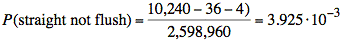or 1 in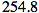g) A three-of-a-kind is three cards showing the same number plus two cards that do not form a pair or create a four-of-a-kind.

If we order the 5-card hand with the three-of-a-kind first, we have 13C1 choices for the number showing on the first three cards. Since we will have three out of four suits, we have 4C3 = 4 ways to choose the suits. The remaining two cards must show different numbers than the four-of-a-kind and each other. There are 12C2 choices for these numbers. The last two cards may have any of the four suits, however.

# 3-of-a-kind = 13C14C312C24C14C1 = 13⋅4⋅66⋅4⋅4 = 54,912

Dividing by the number of possible hands gives the probability: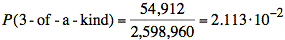or 1 in 47.3.

h) Two-pairs is two cards showing the same numbers and another two cards showing the same numbers (but not all four numbers the same) plus one extra card (not the same as any of the other numbers).

If we order the 5-card hand with the two pairs first, we have 13C2 choices for the two numbers showing on the two pairs. Each pair will have two out of four suits. Thus, we have 4C24C2 = 6⋅6 = 36 ways to choose the suits. The remaining card must show a different number than the two pairs. There are 11C1 choices for this number. The last card may have any of four suits.

# 2-pair = 13C24C24C211C14C1 = 78⋅6⋅6⋅11⋅4 = 123,552

Dividing by the number of possible hands gives the probability: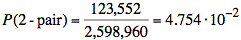or 1 in 21.

i)  One-pair is two cards showing the same numbers and another three cards all showing different numbers.

If we order the 5-card hand with the pair first, we have 13C1 choices for the number showing on the pair. The pair will have two out of four suits. Thus, we have 4C2 = 6 ways to choose the suit. The remaining three cards must show different numbers than the pair and each other. There are 12C3 choices for these numbers. The last three cards may each have any of four suits.

# 1-pair = 13C14C212C34C14C14C1 = 13⋅6⋅220⋅4⋅4⋅4 = 1,098,240

Dividing by the number of possible hands gives the probability: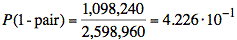or 1 in 2.37.

j)  High card means we must avoid higher-ranking hands. All higher-ranked hands include a pair, a straight, or a flush.

We now count the number of possible high-card hands

Because the numbers showing on the cards must be five different numbers, we have 13C5 choices for the five numbers showing on the cards. Each of the cards may have any of four suits.

13C54C14C14C14C14C1 = 1287⋅45 = 1,317,888

We subtract the number of straights, flushes, and royal flushes. (Note that we avoided having any pairs or more of a kind.) Dividing the difference just calculated by the number of possible hands gives the probability: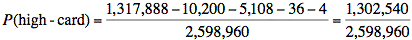or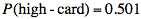or 1 in 2.

Ref:    Probability: 5-card Poker Hands, Tom Ramsey, http://www.math.hawaii.edu/~ramsey/Probability/PokerHands.html, Feb. 1, 2008.